1. 顺序查找，也叫线性查找
2. 二分查找，也叫折半查找
3. 插值查找
4. 斐波那契查找

# 1.顺序查找

public class seqSearch {
public static void main(String[] args) {
//定义数组arr
int[] arr = {1,8, 10, 89, 1000, 1234};
//定义下标
int index = seqSearch(arr, 8);
//当下标为-1时，说明要查找的数不存在
if (index == -1) {
System.out.println("不存在");
} else {
System.out.println("下标为：" + index);
}
}
public static int seqSearch(int[] arr, int value) {
for (int i = 0; i < arr.length; i++) {
if (arr[i] == value ) {
return i;
}
}
//因为下标数值总是>= 0,所以，当要查找的数不存在时，返回-1即可
return -1;
}
}


import java.util.ArrayList;

public class seqSearch {
public static void main(String[] args) {
int[] arr = {1, 9, 11, 11, 52, 65, -4};
ArrayList<Integer> arrayList = seqSearch(arr, 11);
//当没有查找到时，则arrayList中则没有下标值
System.out.println("arrayList：" + arrayList);
}

public static ArrayList<Integer> seqSearch(int[] arr, int value) {
ArrayList<Integer> arrayList = new ArrayList<Integer>();

for (int i = 0; i < arr.length; i++) {
if (arr[i] == value ) {
//将满足条件的下标放入arrayList中
}
}
return arrayList;
}
}



# 2.二分查找

1. 我们要新建一个有序数组arr
2. 确定该数组中间值的下标 mid = (left + right) / 2;
3. 让需要查找的数findVal 和中间值 arr[mid] 比较
3.1 findVal > arr[mid], 说明你要查找的数在mid的右边，因此需要 递归的向查找
3.2 findVal < arr[mid], 说明你要查找的数在mid的左边，因此需要 递归的向查找
3.3 findVal = arr[mid], 说明你找到了，就返回mid
4. 既然我们使用了 递归，那么什么时候我们结束递归呢？
4.1 找到就结束递归
4.2 递归完整个数组，仍然没有找到findVal，也需要结束递归。当 left > right 则说明整个数组递归完了，退出。

public class BinarySearch {
public static void main(String[] args) {
int[] arr = {1, 2, 3, 4, 5, 6, 7, 8, 9};
//注意： right 最大值 取不到 arr.length， 最大是arr.length - 1
int resIndex = binarySearch(arr,0, arr.length - 1,8);
System.out.println("resIndex: " + resIndex);
}

//二分查找
/**
* @param arr  		 有序数组
* @param left		 序列左边
* @param right		 序列右边
* @param findVal    要找的数
* @return 			 如果找到就返回下标，否则返回 -1
*/
public static int binarySearch(int[] arr, int left, int right, int findVal) {
//当left > right 时， 说明递归整个数组， 但是没有找到
if (left > right) {
return -1;
}
int mid = (left + right) / 2;
int midVal = arr[mid];

//向右递归
if (findVal > midVal) {
return binarySearch(arr, mid + 1, right, findVal);
} else if (findVal < midVal) {
//向左递归
return binarySearch(arr, left,mid - 1, findVal);
} else {
return mid;
}
}
}



import java.util.ArrayList;

/**
*    问题：
*          {1, 2, 3, 4, 5, 6, 7, 8, 8, 9}  当一个有序数组中，有多个相同的数值时，
*    如何将所有的数值都查找到，比如这里的 8.
*/
public class BinarySearch2 {
public static void main(String[] args) {
int[] arr = {1, 2, 3, 4, 5, 6, 7, 8, 8, 9};
ArrayList<Integer> resIndexList = binarySearch2(arr, 0, arr.length - 1, 8);
System.out.println("resIndexList = " + resIndexList);
}

public static ArrayList binarySearch2(int[] arr, int left, int right, int findval) {
//如果left > right , 说明递归了整个数组，退出循环
if (left > right) {
return new ArrayList<Integer>();
}

int mid = (left + right) / 2;
int midVal = arr[mid];

//向右循环
if (findval > midVal) {
return binarySearch2(arr, mid + 1, right, findval);
} else if (findval < midVal) {
//向左循环
return binarySearch2(arr, left, mid - 1, findval);
} else {
//         思路：
//              1. 在找到mid 索引值后，不要立刻返回
//              2. 向mid 索引值的左边扫描，将所有等于 8 的元素的下标，加入到集合ArrayList
//              3. 向mid 索引值的右边扫描，将所有等于 8 的元素的下标，加入到集合ArrayList
//              4. 将ArrayList 返回

ArrayList<Integer> resIndexList = new ArrayList<Integer>();

//向mid 索引值的左边扫描，将所有等于 8 的元素的下标，加入到集合ArrayList
int temp = mid - 1;
while (true) {
if (temp < 0 || arr[temp] != findval) {
break;
}
//否则，就temp 放入resIndexList
//temp 左移
temp--;
}
//将中间的mid 下标放入

//向mid 索引值的右边扫描，将所有等于 8 的元素的下标，加入到集合ArrayList
temp = mid + 1;
while (true) {
if (temp > arr.length - 1 || arr[temp] != findval) {
break;
}
//否则，就temp 放入resIndexList
//temp 右移
temp++;
}
return resIndexList;
}
}
}



# 3. 插入查找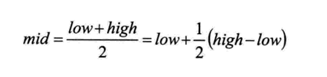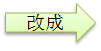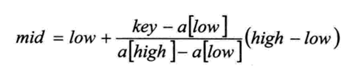import java.util.ArrayList;
import java.util.Arrays;

public class InsertValueSearch {
//count 表示递归次数
private static int count = 0;

public static void main(String[] args) {
//定义一个有100个元素的数组
int arr[] = new int;
for (int i = 0; i < arr.length; i++) {
arr[i] = i;
}
//将数组转成字符串输出
System.out.println(Arrays.toString(arr));

ArrayList<Integer> resArrayList = insertValueSearch(arr, 0, arr.length - 1, 8);
System.out.println("resArrayList = " + resArrayList);
System.out.println("递归次数：" + count);

}

//插值查找
public static ArrayList insertValueSearch(int[] arr, int left, int right, int findVal) {
count++;
//如果left > right,则说明已经递归完数组中所有的数字， 退出
//findval < arr || findval > arr[arr.length - 1] 必须要有，因为当findVal非常大时，会导致mid越界
if (left > right || findVal < arr || findVal > arr[arr.length  - 1]) {
return new ArrayList<Integer>();
}

//找到中间值
int mid = left + (right - left) * (findVal - arr[left]) / (arr[right] - arr[left]);
int midVal = arr[mid];

//向左递归
if (findVal < midVal) {
return insertValueSearch(arr, left, mid - 1, findVal);
} else if (findVal > midVal) {
//向右递归
return insertValueSearch(arr, mid + 1, right, findVal);
} else {

ArrayList<Integer> resArrayList = new ArrayList<Integer>();
//往mid 左边扫描,将所有等于 8 的元素的下标，加入到集合ArrayList
int temp = mid - 1;
while (true) {
//temp < 0 则意味着，mid在数组的最左边
if (temp < 0 || arr[temp] != findVal) {
break;
}
//左移一位
temp--;
}
//将中间的mid 下标放入

//往mid 右边扫描,将所有等于 8 的元素的下标，加入到集合ArrayList
temp = mid + 1;
while (true) {
//temp > arr.length - 1 则意味着，mid在数组的最右边
if (temp > arr.length - 1 || arr[temp] != findVal) {
break;
}
//右移
temp++;
}
return resArrayList;
}
}
}



1. 对于数据量较大，关键字分布比较均匀的查找表来说，采用插值查找，速度较快。
2. 关键字分布不均匀的情况下，该方法不一定比折半查找好。

PS:关于插值查找算法的mid值为什么要这么求，我也不是很清楚。不过我认为如果你不是专门做算法的，那咱们知道应该在何时何地使用这种算法就可以了。

# 4.斐波那契查找算法

• 斐波那契(黄金分割法)查找基本介绍:
1. 黄金分割点是指把一条线段分割为两部分，使其中一部分与全长之比等于另一部分与这部分之比。取其前三位数字的近似值是0.618。由于按此比例设计的造型十分美丽，因此称为黄金分割，也称为中外比。这是一个神奇的数字，会带来意向不大的效果。
2. 斐波那契数列 {1, 1, 2, 3, 5, 8, 13, 21, 34, 55 } 发现斐波那契数列的两个相邻数 的比例，无限接近 黄金分割值0.618
• 斐波那契(黄金分割法)查找算法：

1. 斐波那契查找原理与前两种相似，仅仅 改变了中间结点（mid）的位置，mid不 再是中间或插值得到，而是位于黄金分 割点附近，即mid=low+F(k-1)-1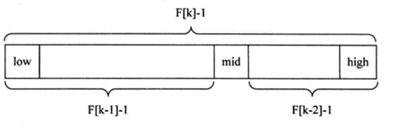1. 对F(k-1)-1的理解：
• 由斐波那契数列 F[k]=F[k-1]+F[k-2] 的性质，可以得到 （F[k]-1）=（F[k-1]-1）+（F[k-2]-1）+1 。该式说明：只要顺序表的长度为F[k]-1，则可以将该表分成长度为F[k-1]-1和F[k-2]-1的两段，即如上图所示。从而中间位置为mid=low+F(k-1)-1
• 类似的，每一子段也可以用相同的方式分割
• 但顺序表长度n不一定刚好等于F[k]-1，所以需要将原来的顺序表长度n增加至F[k]-1。这里的k值只要能使得F[k]-1恰好大于或等于n即可，由以下代码得到,顺序表长度增加后，新增的位置（从n+1到F[k]-1位置），都赋为n位置的值即可。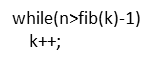• 举例说明： 请对一个有序数组进行斐波那契查找 {1,8, 10, 89, 1000, 1234} ，输入一个数看看该数组是否存在此数，并且求出下标，如果没有就提示"没有这个数"。
import java.util.Arrays;

public class FibonacciSearch {
//斐波那契数组元素的个数，
public static int maxSize = 20;
public static void main(String[] args) {
int[] arr = {1, 8, 10, 89, 1000, 1234};
System.out.println(fibSearch(arr,89));

}

//因为后面我们mid = low + F(k-1) - 1,需要使用到斐波拉契数列，因此我们需要先获取一个斐波那契数列
//非递归方法得到斐波那契数列
public static int[] fib() {
int[] f = new int[maxSize];
f = 1;
f = 1;
for (int i = 2; i < maxSize; i++) {
f[i] = f[i - 1] + f[i - 2];
}
return f;
}

//编写斐波那契查找算法
//非递归的方式编写斐波那契查找算法
/**
*
* @param a     数组
* @param key   我们需要查找的关键码
* @return      返回对应的下标，如果没有就返回 -1
*/
public static int fibSearch(int[] a, int key) {
int low = 0;
int high = a.length - 1;
//表示斐波那契分割数值的下标
int k = 0;
int mid = 0;

//获取到斐波那契数列
int f[] = fib();
//获取到斐波那契分割数值的下标
while (high > f[k] - 1) {
k++;
}
//因为f[k] 值可能大于我们数组a 的长度，因此我们需要使用Array类，构造一个新的数组，并指向a
//不足的部分会使用0填充
int[] temp =  Arrays.copyOf(a, f[k]);
//实际上需要使用a数组最后的数填充temp
/**
*  举例说明：
*      int[] arr = {1, 8, 10, 89, 1000, 1234};
*      int[] temp =  Arrays.copyOf(a, f[k]);
*      当 数组a 的长度 小于 f[k] 的长度， 我们要将a 的值赋值给数组temp ，但是
*      数组temp 的长度是和f[k] 相同的，所以 数组temp 中没有被赋值的部分 填充为0；
*      又因为，如果数组temp 中没有被赋值的部分
*
*/
for (int i = high + 1; i < temp.length; i++) {
temp[i] = a[high];
}

//使用while来循环处理，找到我们的数key
while (low <= high) {
//只要这个条件满足，就可以继续寻找
//得到mid 中间值 的规则 不一样
mid = low + f[k - 1] - 1;

//向左边查找
if (key < temp[mid]) {
high = mid - 1;
/*
为什么是k--
1.  全部元素 = 前面的元素 + 后面元素
2.  f[k] = f[k - 1] + f[k - 2]
因为 前面f[k - 1] 个元素，所以可以继续拆分 f[k-1] = f[k-2] + f[k-3]
即在f[k-1] 的前面继续查找 k--
即，下次循环 mid = f[k-1-1] -1
*/
//重点！！！！难点！！！
k--;
} else if (key > temp[mid]) {
//右边查找
low = mid + 1;
/*
为什么是 k -= 2
1. 全部元素 = 前面的元素 + 后面元素
2. f[k] = f[k - 1] + f[k - 2]
3. 因为后面我们有f[k - 2] 所以可以继续拆分 f[k-2] = f[k-3] + f[k-4]
4. 即在f[k-2] 的前面 进行查找 k -= 2
5. 即下次循环 mid = f[k - 1 - 2] - 1
*/
//重点！！！！难点！！！
k -=2;
} else {
//找到了
//需要确定返回的是哪个下标
if (mid <= high) {
return mid;
} else {
return high;
}
}
}
return  -1;
}
}



posted @ 2019-06-21 17:40  行走在代码边缘  阅读(284)  评论(0编辑  收藏